# Making a Multi-D Scatter Plot

(diff) ← Older revision | Latest revision (diff) | Newer revision → (diff)

In this tutorial example, we'll plot the points from a 4-D Gaussian distribution as a scatter plot. You will learn how to set up a scatter plot when the coordinates of the data are organized as columns in a single table, and how such plots can be interactively pivoted to view the scatter points from each dimension.

First, let's create the data to be plotted. For this, we'll define a 4-D Gaussian distribution. Follow these steps:

2. In the model's Object window, fill in the title and description.3. Close the Object window.

4. Select File → Add Library... → Multivariate Distributions.ana

5. Create these two indexes:

Index Dim := [1, 2, 3, 4]
Index Dim2 := CopyIndex(Dim)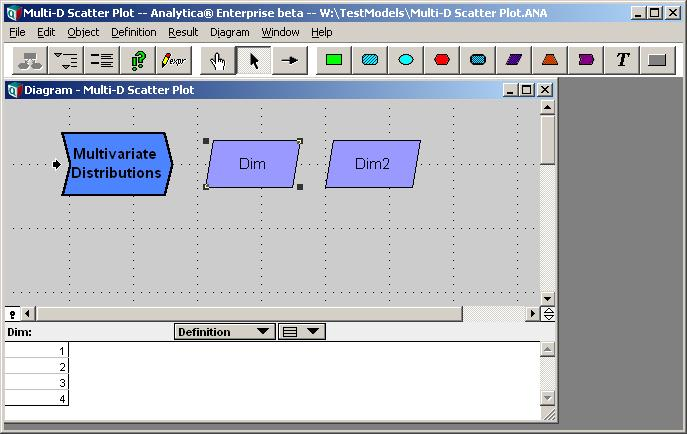7. Define the covariance matrix. Create a variable named covar and set the definition type to Table. Select the Dim and Dim2 indexes and fill in the Edit table with a covariance matrix: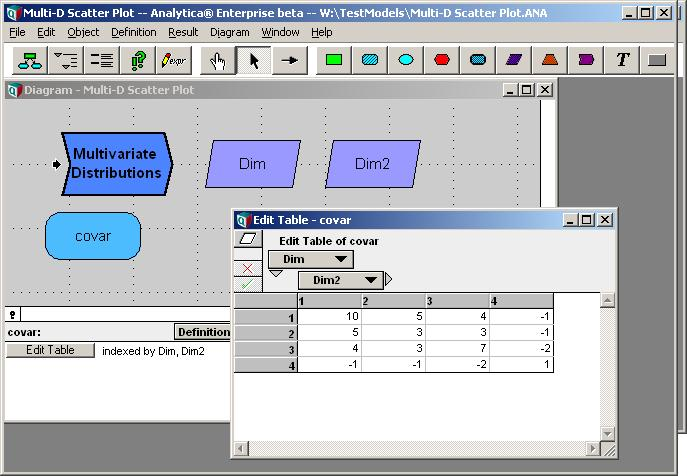8. Define the Gaussian distribution. Create a chance variable node named X and set the definition to:

Gaussian(0, covar, Dim, Dim2)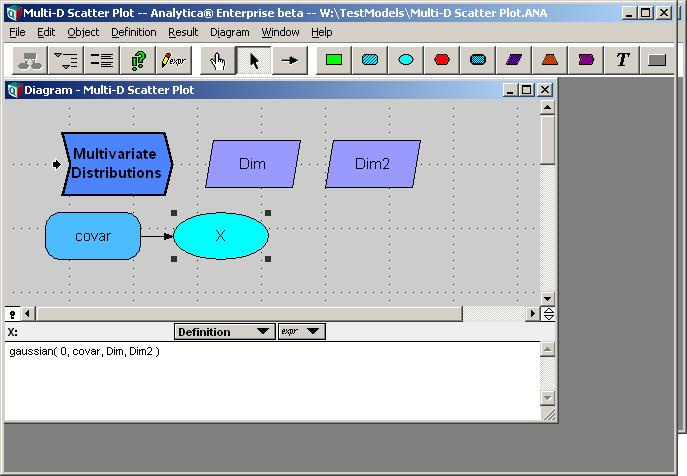9. Select Result → Uncertainty Options... and set the sample size to 1000. (so we have more points on our plot)10. Select X and show Result → Sample. Switch to graph mode if not already.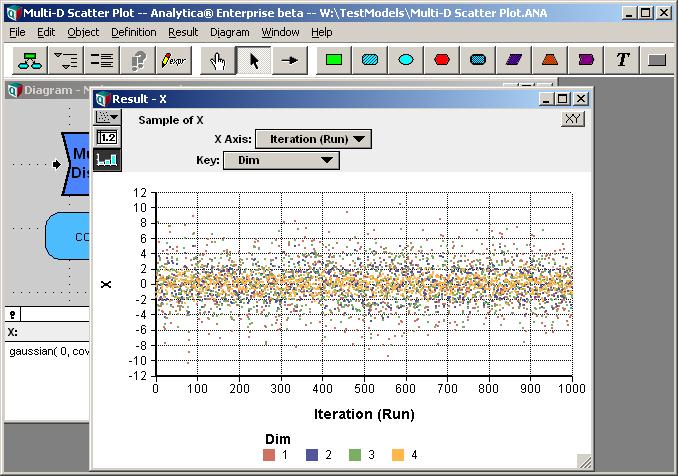11. Switch to table view to examine the actual data. For convenience, pivot so that Index Dim forms the columns, Run the rows.## Setting the Coordinate Index

In the initial plot, Analytica treats the data as four series. What we desire is to treat each row in the above table as a single data point to be plotted. Each point is four-dimensional. Of course, on a 2-D graph, with just an X and Y axis, we will be viewing two coordinates at a time, but we can interactively pivot between the various combinations.

In order to use the columns of the data as the coordinates of each data point, we need to tell Analytica that the Dim index is to be interpreted as the Coordinate Index.

11. Press the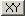at the top-right corner of the result window. In the dialog, select "Use Coordinate Index". Note: You must be in edit mode to make changes in this dialog.12. Close the dialog by pressing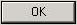There are a few things to notice about the result window now. A coordinate index pulldown appears at the top. Here we see that Analytica is using the Dim index as the coordinate index, as we desire. The horizontal dimension of the table is now "No Index", and the top row of the column headers is blank. The values of Dim are now appears in the second row of column headers. This indicates that Analytica is treating these as four different values (for graphing, these are value dimensions) all sharing a common index (Run). As different values, they can be plotted relationally against each other.

13. Pushto switch to graph mode. Now we have our first scatter plot.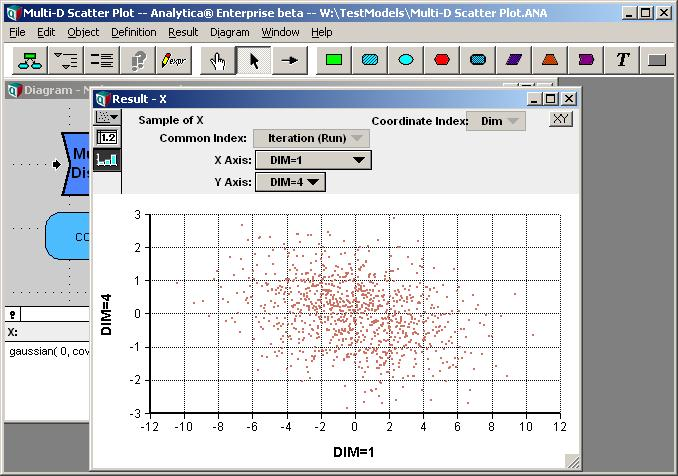14. Change the Y-Axis pivoter to Dim = 2. This shows us another 2-D projection of the same 4-D scatter data. Spend some time selecting different combinations of X-axis and Y-axis values.## Changing the Dimension Labels

In the above graphs, the axis labels display as "Dim = 1", etc., using the name of the index and the value.

Rather than use numeric values for the index, let's switch to something more descriptive.

15. Return to the diagram and edit the definition of Index Dim. Change the definition to a list-of-labels and enter labels in place of the numeric values as follows.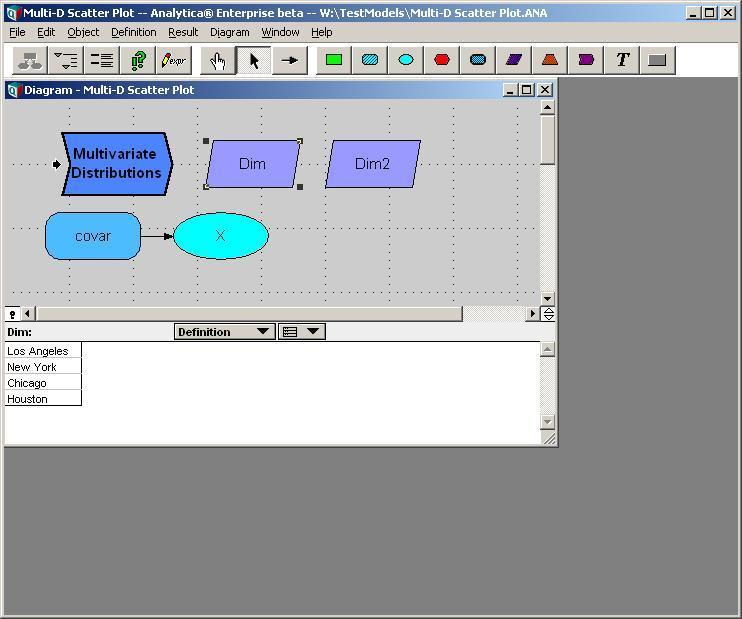16. Redisplay the result graph for X and notice the change in labels.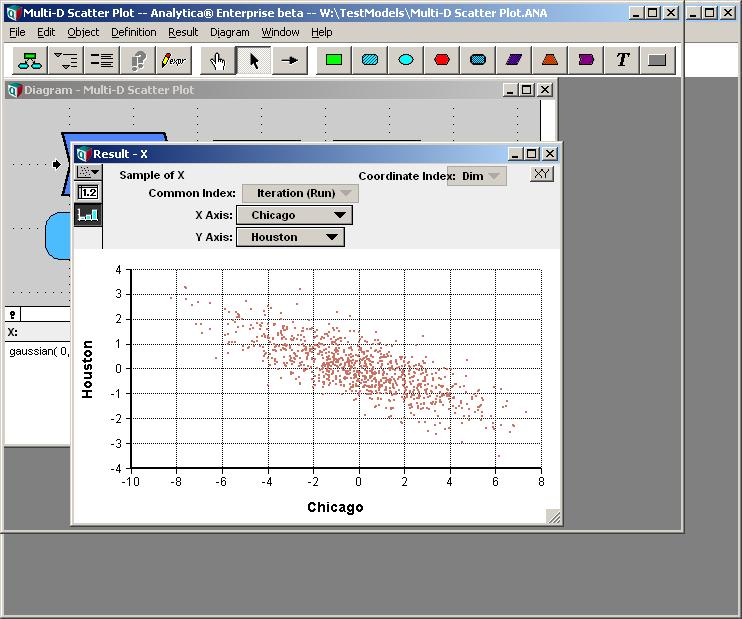## Overlaying Two Scatter Plots

Next, let's overlay two 4-D scatter plots on the same graph. We'll use a 4-D Gaussian again for the second scatter plot data, but with a different covariance and centroid.

17. Set up the covariance matrix for the second scatter data. Create a new variable, name it covar2, define it as a Table with indexes Dim and Dim2, and fill in a covariance matrix as follows.18. We'll also use a non-zero mean (centroid) this time, so set up this. Create a variable, name it m, define it as a table with Index Dim, and fill it is in follows.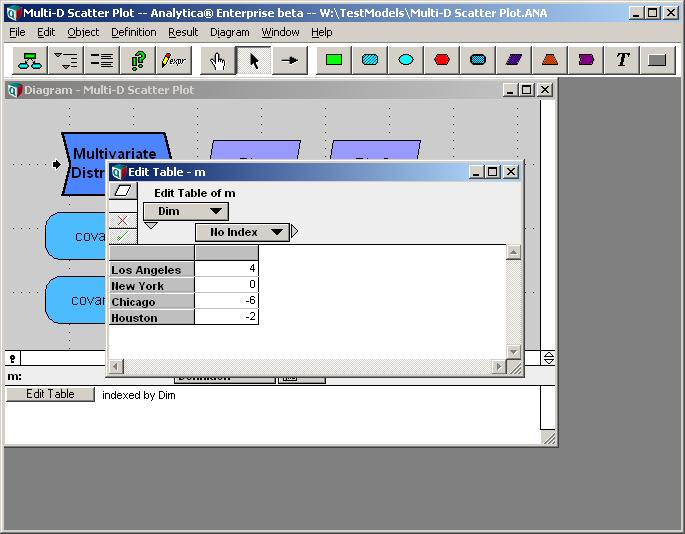19. Next, use the Gaussian function to create the data. Create a new chance variable, Y, and set its definition to

Gaussian(m, covar2, Dim, Dim2)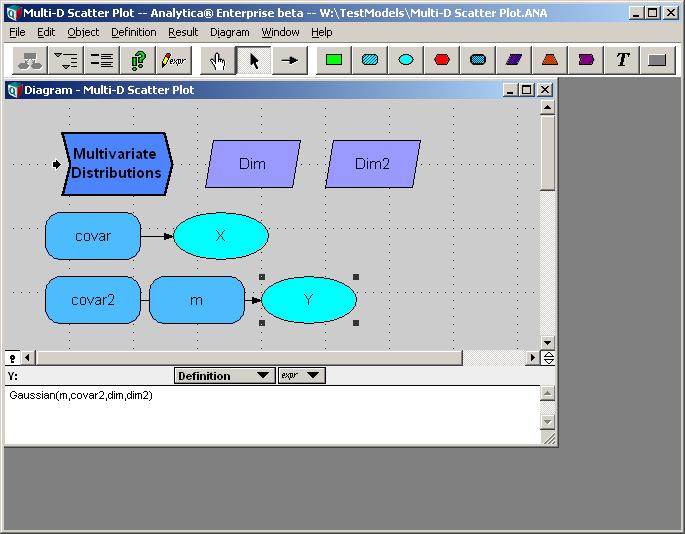At this point, we could plot Y using the steps outlined previous for X. However, the real goal here is to plot X and Y together on the same graph. So, let's bring up their combined result.

20. On the diagram, select both X and Y. To do this, click on X, then hold the shift key down and click on Y. Then on the Result menu, select Sample.

Analytica creates a new variable to hold the combined result, and initially names this Va1. Before proceeding, let's rename it.

21. On the toolbar, click on the Object window button. Change the title from Va1 to Scatters.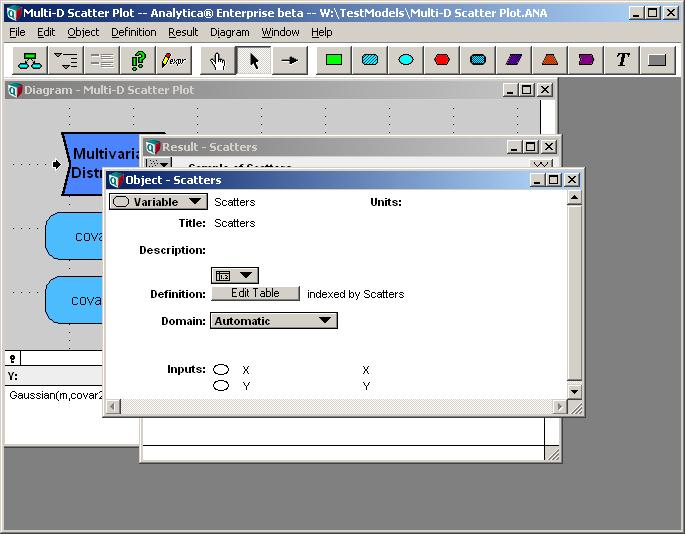22. Close the object window. The result view for Scatter will be in focus again. If you are not already viewing a graph, graph the data by pressing.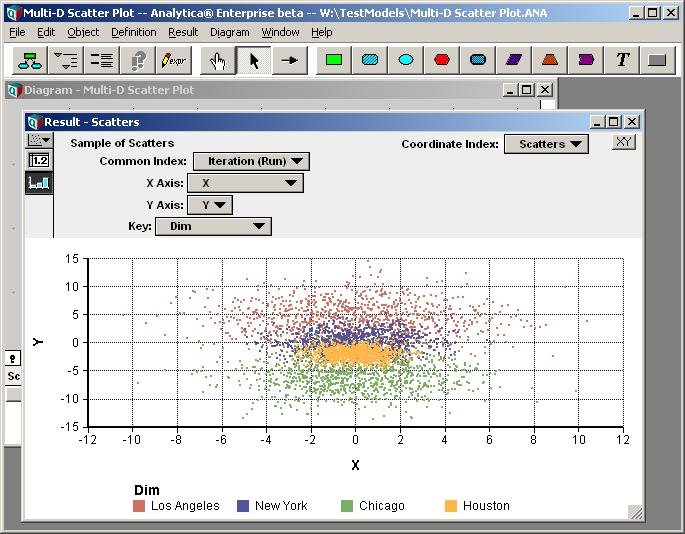The graph that initially displays has the result of X on the X-axis, and the result of Y on the Y-axis. This is not what we desire, but this is being shown because the Scatters index is being used as the Coordinate Index. Scatters is the comparison variable we just set up, its index value contains two elements, X and Y, which serves just fine as a 2-D coordinate.

23. Change the coordinate index pulldown to "Dim".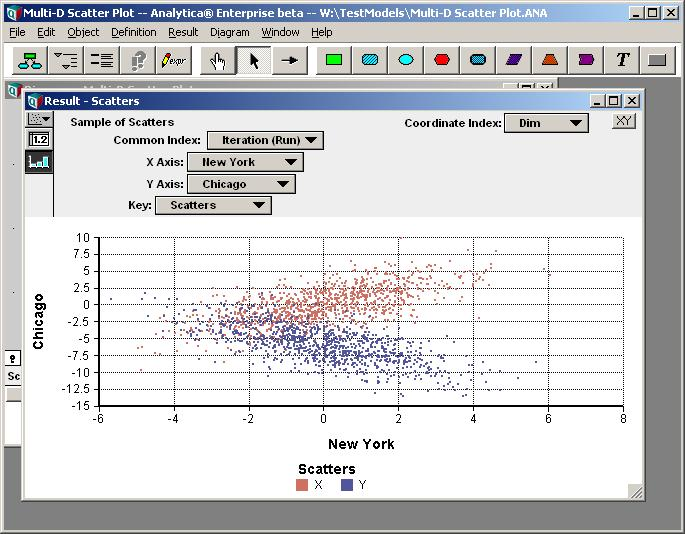Now we have both data sets overlaid on a single scatter plot. The first data set, X, displays in red, the second in blue.